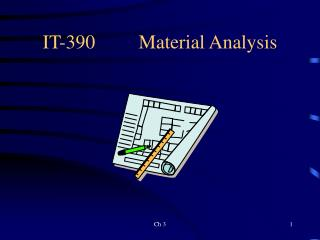DownloadDownload PresentationIT-390 Material Analysis

# IT-390 Material Analysis

Download Presentation## IT-390 Material Analysis

- - - - - - - - - - - - - - - - - - - - - - - - - - - E N D - - - - - - - - - - - - - - - - - - - - - - - - - - -
##### Presentation Transcript

1. Material • Substance being altered or used in an alteration • Purchased (not manufactured) • Materials required for a design - Bill of Materials (BOM) • Part list (type of material and quantity) • Material details • Specifications - reside separately from the BOM

2. Material • Raw, standard commercial items, subcontract items, interdivisional transfer • Raw Materials - Silica (sand) for glass manufacture • Standard Commercial Items - Nuts, bolts, screws, bearings,... • Subcontract Items - produced by an outside manufacturer • Interdivisional Transfer Materials - transferred between divisions of a corporation

3. Material • Engineering, commodity, semi-engineering, and normative materials. • Engineering Materials - former commodities - have been substantially refined, such as aluminum, which is refined from bauxite. • Commodities - basic ores (bauxite, iron ore,...) • Semi-engineering Materials - gray area between commodities and engineering materials. • Normative Materials - price fixed through government intervention or cartels

4. Material • Direct materials - go directly into the end product - come from the BOM. • Indirect materials - necessary for conversion or manufacturing process - do not end up in the final product - (sand paper, cutting oil, cleaning solvents,...)

5. Shape • Different approaches to Direct material estimation • How much material (in weight or volume) - cost

6. Shape • Theoretical approach • Appropriate for an injection molded plastic part or a die cast aluminum part • Allowances - Scrap, Waste, and Shrinkage. • Scrap - faulty material - human error • Waste - by-product of the manufacturing or conversion process • Shrinkage - loss of material due to physical laws or theft (aging, oxidation, chemical reaction, spoilage,.)

7. Shape • Second approach • Solid chunk of material - make mathematical subtractions • Both approaches calculate the "shape" using geometry/trigonometry • Shape - implies mass, area, or length - not the physical outline

8. Shape • If complicated, cut it up and calculate separately, summing the results • Cost of direct - reduced slightly by ability to sell waste material or recycle • Sawdust

9. Shape • Efficiency- raw material - finished product • In this case, the shape yield could be calculated from formula 3.2 on page 88: • Es = St/Sa x 100% • Where: Es = shape yield • Sa = actual (original) shape in units of area, length, mass, volume, count, etc. • St = theoretical (final) shape required for design in units of area, length, etc.

10. Problem 3.19 a (pg. 120) What is the number of bars required? We will assume that both ends of the bar are faced From one bar we can cut: 144” – 6” = Bar length – Gripping = 8.36 units/bar 16”+1/16+1/16+3/8 Part length + Facing + Cutoff  8 units/bar Units required = 260 + 1% (scrap) + ½ % (shrinkage) = 264 units (260x.01 = 2.6, and 260x.005 = 1.3) Number of bars required = 264 / 8 = 33 bars required

11. Problem 3.19 b (pg. 120) What is the shape yield, Es? Es = St/Sa x 100% or Es = theoretical shape/actual shape x 100% Must calculate based on all =260(1)² (16”) x (100%) = 87.5% parts and all bars required! 33(1)² (144”) Remember, area of cylinders are expressed as R²

12. Problem 3.19 c (pg. 120) What is the material cost per piece? Remember: part cost must not just absorb the part itself, but the waste also! Part Cost = Cost of bars / Total parts required =33 bars ( Cost per bar) 260 parts Part Cost = 33(96.02)=\$12.19/unit 260 -------- Cost = (area)(length)(density)(cost/lb) Bar Cost = (1 in.)² (144 in.) (.283 lb/in³) (\$.75/lb) = \$96.02/bar 144”  R² 2” dia.

13. Handout Example Problem • A part is machined as shown in the handout. The original stock size of AISI 1045 material is 5 X 2.5 X 2 in. Density = 0.29 lb/in3. • If this material will cost \$1.90/lb, then what is the unit cost of the raw material. (Before processing) • What cost is lost to waste given that the waste is salvaged at 10% of original value? • Find the shape yield.